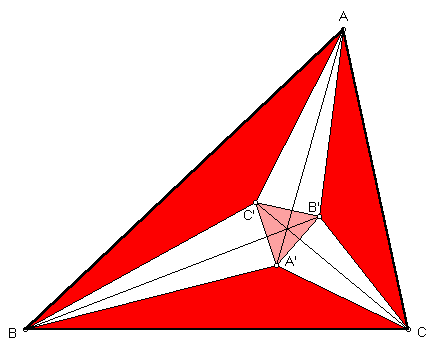# 1ST AND 2ND MORLEY CENTERS

The famous Morley Theorem states that the three points of intersection of the adjacent trisectors of the angles of a triangle ABC form an equilateral triangle. In 1967, Peter Yff proved that the center of the Morley triangle, called the 1st Morley center, is given by trilinear coordinates

cos(A/3) + 2 cos(B/3) cos(C/3) :
cos(B/3) + 2 cos(C/3) cos(A/3) :
cos(C/3) + 2 cos(A/3) cos(B/3).

#While studying the 1st Morley Center, Peter Yff proved that the Morley equilateral triangle is perspective to triangle ABC, and that the center of perspective, or 2nd Morley center, has remarkably simple trilinears:

sec(A/3) : sec(B/3) : sec(C/3)

For an introduction to the Morley triangles and 150 further references on these remarkable objects, see

Cletus O. Oakley and Justine C. Baker, "The Morley trisector theorem," Amer. Math. Monthly 85 (1978) 737-745.

(For a quick lesson on trilinears, click on TRILINEAR COORDINATES.)
Biographical Sketch of Frank Morley
Triangle Centers